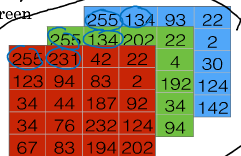### 图片在深度学习中的常用表示$x=\begin{bmatrix} x_1 \\ x_2 \\ x_3 \end{bmatrix}$

$x=\begin{bmatrix} 255 \\ 231 \\ ... \\ 255 \\ 134 \\ ... \\ 255 \\ 134 \end{bmatrix}$

### 课程中常用的符号约定

• 输入、输入元组: $(x, y)$，其中$x \in \mathbb{R}^{n_x}$,$y \in\mathbb{R}$
$x$是一个$n_x$维的特征向量，$y$是1维输出
• 训练集由m个样本组成，则训练样本依次表示为:
$m= m_{train}= \{(x^{(1)}, y^{(1)}), (x^{(2)}, y^{(2)}) ... (x^{(m)},y^{(m)})\}$，测试集$m= m_{test}$的表示同理
• 将训练集表示成矩阵:
$X = \begin{bmatrix} | & | & ... & | \\ x^{(1)} & x^{(2)} & ... & x^{(m)} \\ | & | & ... & | \end{bmatrix}$
$X \in \mathbb{R}^{n_x \times m}$，即$X$是一个$n_x \times m$维的矩阵
输出:
$Y = \begin{bmatrix} y^{(1)} & y^{(2)} & ... & y^{(m)} \end{bmatrix}$
$Y \in \mathbb{R}^{1 \times m}$，即$Y$是一个$1 \times m$维的矩阵